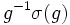# Unipotent automorphism

Let$G$ be a group. For$\sigma \in \operatorname{Aut}(G)$ and$g \in G$, denote by$[g,\sigma]$ the element$g^{-1}\sigma(g)$. Then$\sigma$ is said to be unipotent of class$n$ if for any$g \in G$:$[[[\ldots[g,\sigma],\sigma],\ldots] = e$
with$\sigma$ written$n$ times.